# Chemical EquilibriumPage 1

#### WATCH ALL SLIDES

Slide 1## Chemical equilibrium

Cato Maximilian Guldberg and his brother-in-law Peter Waage developed the Law of Mass Action

Slide 2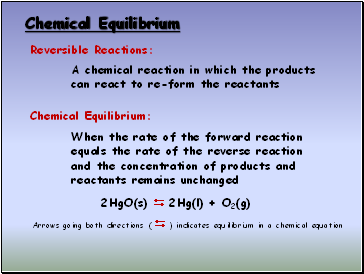Chemical Equilibrium

Reversible Reactions:

A chemical reaction in which the products

can react to re-form the reactants

Chemical Equilibrium:

When the rate of the forward reaction

equals the rate of the reverse reaction

and the concentration of products and

reactants remains unchanged

2HgO(s)  2Hg(l) + O2(g)

Arrows going both directions (  ) indicates equilibrium in a chemical equation

Slide 3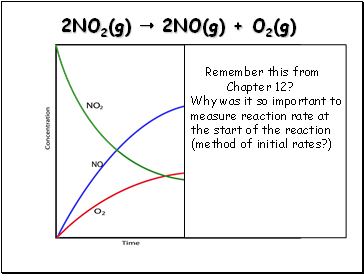2NO2(g)  2NO(g) + O2(g)

Remember this from

Chapter 12?

Why was it so important to measure reaction rate at the start of the reaction

(method of initial rates?)

Slide 4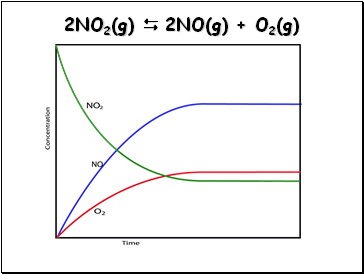2NO2(g)  2NO(g) + O2(g)

Slide 5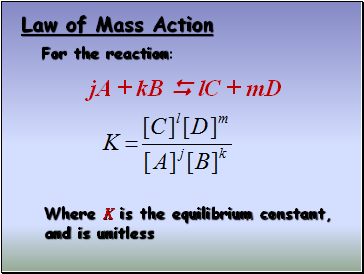## Law of Mass Action

For the reaction:

Where K is the equilibrium constant, and is unitless

jA + kB  lC + mD

Slide 6## Product Favored Equilibrium

Large values for K signify the reaction is “product favored”

When equilibrium is achieved, most reactant has been converted to product

Slide 7## Reactant Favored Equilibrium

Small values for K signify the reaction is “reactant favored”

When equilibrium is achieved, very little reactant has been converted to product

Slide 8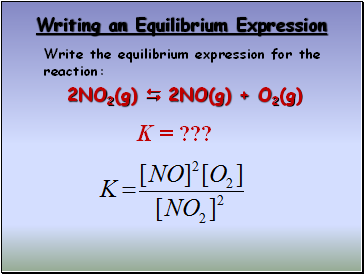## Writing an Equilibrium Expression

2NO2(g)  2NO(g) + O2(g)

K = ???

Write the equilibrium expression for the reaction:

Slide 9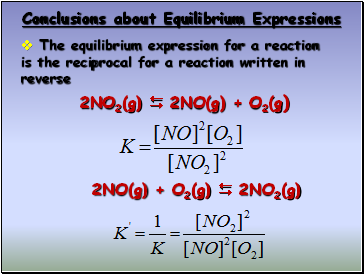The equilibrium expression for a reaction is the reciprocal for a reaction written in reverse

2NO2(g)  2NO(g) + O2(g)

2NO(g) + O2(g)  2NO2(g)

Slide 10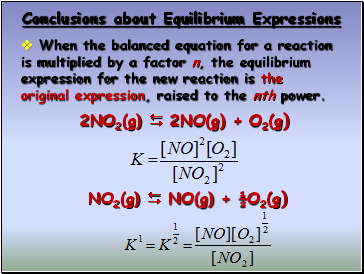When the balanced equation for a reaction is multiplied by a factor n, the equilibrium expression for the new reaction is the original expression, raised to the nth power.

2NO2(g)  2NO(g) + O2(g)

NO2(g)  NO(g) + ½O2(g)

Slide 11Go to page:
1  2